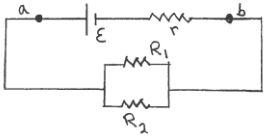# Problem: The circuit shown in the sketch consists of a battery with emf ε and internal resistance r connected to two resistors as shown. R1 = 3.0 Ω, R2 = 6.0 Ω and r = 0.50 Ω. The terminal voltage Vab of the battery is 14.0 V. At what rate is electrical energy dissipated in  R2?

###### FREE Expert Solution
84% (264 ratings)
###### Problem Details

The circuit shown in the sketch consists of a battery with emf ε and internal resistance connected to two resistors as shown. R1 = 3.0 Ω, R2 = 6.0 Ω and r = 0.50 Ω. The terminal voltage Vab of the battery is 14.0 V. At what rate is electrical energy dissipated in  R2?